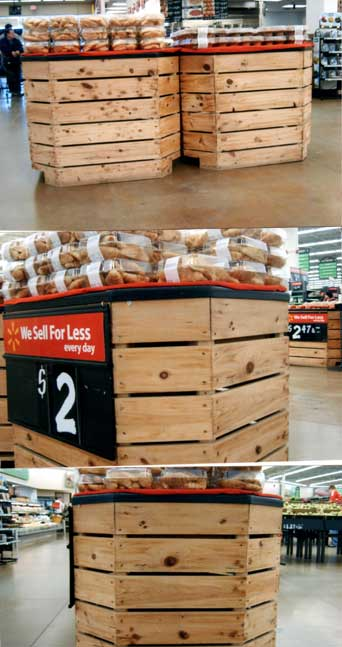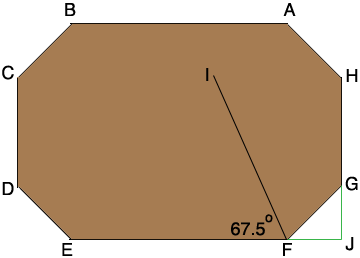SEARCH HOMEMath Central Quandaries & QueriesQuestion from Gayle: I am building an irregular shaped octagon wooden box. The measurements are 291/2 inches by 211/2 inches. Sides are 12 inches. It will be 36 inches high. What would the cutting angles degrees be?Gayle,

There is not enough information in your email to calculate the angles. Can you send a diagram?

Harley

Gayle sent these photos.Gayle,

Looking down from the top the octagon I see is (not to scale)I added labels, drew FI which bisects angle EFG and extended the sides EF and HG to meet at J. If the sides AB and EF are parallel and the sides CD and GH are parallel, as they seem to be in your pictures, and if the lengths FJ and JG are equal then the angle GFJ measures 45o. This hen means that the angle EFG measures 180o - 45o = 135o and thus angle EFI measures 135o/2 = 67.5o. This is the cutting angle.

HarleyMath Central is supported by the University of Regina and The Pacific Institute for the Mathematical Sciences.